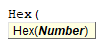# VBA Hex Function

## Hex Description

Converts a numeric value to hexadecimal notation and returns the result as a string.

## Hex Syntax

In the VBA Editor, you can type  “Hex(” to see the syntax for the Hex Function:The Hex function contains an argument:

Number: the numeric value that you want to convert to hexadecimal.

## Examples of Excel VBA Hex Function

``MsgBox Hex(10)``

Result: A

``MsgBox Hex(15)``

Result: F

``MsgBox Hex(255)``

Result: FF

``MsgBox Hex(&O12)``

Result: A

``MsgBox Hex(&O77)``

Result: 3F

Stop searching for VBA code online. Learn more about AutoMacro - A VBA Code Builder that allows beginners to code procedures from scratch with minimal coding knowledge and with many time-saving features for all users!